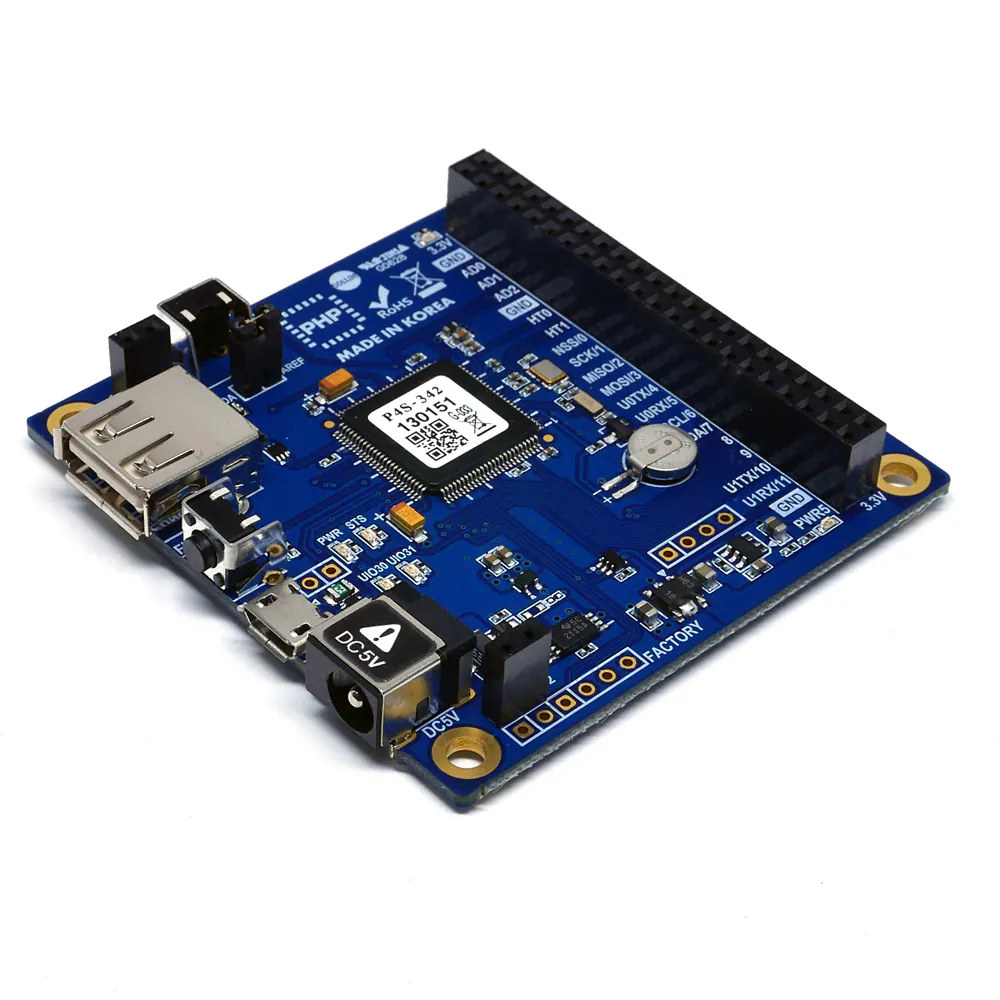# PHPoC - Scratch Programming to Control Robot

Let's "scratch" with PHPoC. Have fun programming a spider robot controller just by dropping some blocks!

BeginnerFull instructions provided1 hour682

## Things used in this project

### Hardware componentsPHPoC Blue
×1
 Spider Robot
×1
 Battery In this project, I use a 3.7V - 2.5A battery as a power source. You can choose other power sources with higher voltage (around 5V), which are suitable for both PHPoC Blue and DC Motor Controller.
×1PHPoC DC Motor Controller (S-type or T-type)
×1

### Software apps and online services

 Scratch Editor 2.0 Offline A free educational platform developed by MIT Media Lab's Lifelong Kindergarten group
 Node.js

## Code

PHP
This script is used to process data and execute commands on PHPoC board.
```<?php

if(_SERVER("REQUEST_METHOD"))
exit; // avoid php execution via http request

include_once "/lib/sd_340.php";
include_once "/lib/sn_tcp_ws.php";
include_once "/lib/sd_spc.php";

define("RC_SPIDER_SID" , 14);
define("WIDTH_MIN", 420);
define("WIDTH_MAX", 1000);
define("PWM_PERIOD", 1000);
define("WS_ID", 0);
define("WS_PATH", "scratch_test");

function dc_init()
{
spc_reset();
spc_sync_baud(115200);
}

function dc_pwm_width(\$dc_id, \$pwm_width, \$pwm_period, \$pol_dir = "+")
{
if((\$dc_id < 1) || (\$dc_id > 2))
exit("dc_pwm_width: dc_id out of range \$dc_id\r\n");

spc_request_dev(RC_SPIDER_SID, "dc\$dc_id pwm set pol \$pol_dir");
spc_request_dev(RC_SPIDER_SID, "dc\$dc_id pwm set period \$pwm_period");
spc_request_dev(RC_SPIDER_SID, "dc\$dc_id pwm set width \$pwm_width");

}

// Define Digital Input ports
for(\$i=12; \$i<=19;\$i++){
uio_setup(0, \$i, "in_pu");
}

// Define Digital Output ports
uio_setup(0, 8, "out high");
uio_setup(0, 9, "out high");
uio_setup(0, 30, "out high");
uio_setup(0, 31, "out high");

ws_setup(WS_ID, WS_PATH, "csv.phpoc");
dc_init();
\$rwbuf = "";

while(1)
{
if(ws_state(WS_ID) == TCP_CONNECTED)
{

if(\$rlen)
{
\$cmd = explode("/",\$rwbuf);
switch(\$cmd){
case "dW":
if (\$cmd == "LOW")
uio_out(0, (int)\$cmd, LOW);
else
uio_out(0, (int)\$cmd, HIGH);
break;
case "poll":
\$ret = "";
for(\$pin=12; \$pin<=19;\$pin++){
\$d_in = uio_in(0, (int)\$pin);
\$ret = \$ret."dR/".(string)\$pin." ".(string)\$d_in."\n";
}
for(\$pin=0; \$pin<=5;\$pin++){
}
ws_write(0, \$ret);
usleep(200000);
break;
case "reset_all":
uio_out(0, 8, HIGH);
uio_out(0, 9, HIGH);
uio_out(0, 30, HIGH);
uio_out(0, 31, HIGH);
dc_pwm_width(1,	0, PWM_PERIOD);
dc_pwm_width(2,	0, PWM_PERIOD);
break;

case "dcwW":
\$pwm_width = (int)\$cmd;
if ((\$pwm_width>=0)&&(\$pwm_width<=PWM_PERIOD)){
dc_pwm_width((int)\$cmd, \$pwm_width, PWM_PERIOD, \$cmd);
}
break;
case "dcpW":
\$pol_dir = "+";
if (\$cmd == "CCW")
\$pol_dir = "-";
\$percent = (int)\$cmd;
if ((\$percent>=0)&&(\$percent<=100)){
dc_pwm_width((int)\$cmd, (int)round(PWM_PERIOD * \$percent/100), PWM_PERIOD, \$pol_dir);
}
break;
case "srW":
switch(\$cmd)
{
case "Stay":
dc_pwm_width(1,	0, PWM_PERIOD);
dc_pwm_width(2,	0, PWM_PERIOD);
break;
case "Go":
dc_pwm_width(1,	800, PWM_PERIOD, "+");
dc_pwm_width(2,	800, PWM_PERIOD, "-");
break;
case "Turn right":
dc_pwm_width(1,	800, PWM_PERIOD, "-");
dc_pwm_width(2,	800, PWM_PERIOD, "-");
break;
case "Turn left":
dc_pwm_width(1,	800, PWM_PERIOD, "+");
dc_pwm_width(2,	800, PWM_PERIOD, "+");
break;
case "Back":
dc_pwm_width(1,	800, PWM_PERIOD, "-");
dc_pwm_width(2,	800, PWM_PERIOD, "+");
break;
default:
dc_pwm_width(1,	0, PWM_PERIOD);
dc_pwm_width(2,	0, PWM_PERIOD);
break;
}
break;
}
}
else {
dc_pwm_width(1,	0, PWM_PERIOD);
dc_pwm_width(2,	0, PWM_PERIOD);
}
}
else {
dc_pwm_width(1,	0, PWM_PERIOD);
dc_pwm_width(2,	0, PWM_PERIOD);
}
}

?>
```

## Credits

### Homer

17 projects • 34 followers